Select Page

# Mass coefficient

### Definition in words

1. Mass of component B divided by coefficient of component B in system 1
2. Mass of component F divided by coefficient of component G in system 1

Note 1: Coefficient can be a kind-of-quantity that is not volume or mass

For the second definition, the following notes apply:

Note 2: Component B is the quotient of component F and component G

Note 2: Component F ≠ Component G (≠ = “not equal to”)

Note 3: Component F ∉ Component G (∉ = “is not an element of”)

### Definition by equation

1.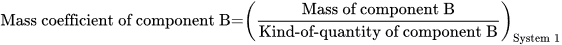2.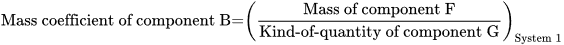### Systematic terms

Mass coefficient of component B in system 1

### Result type

Rational scale
• Value sets are positive numerical values
• Algebraic operations are allowed (a=nb; a≠nb)
Numerical values from 0-∞

### Unit

The corresponding unit depends on the chosen coefficient (denominator kind-of-quantity). The numerator unit can be µg, mg, kg, etc.

For mass coefficient (mass/amount-of-substance), the corresponding unit can e.g. g/mol.

### Special rule(s) in the NPU syntax

The coefficient must be specified in the specification to kind-of-property.

### Reference

Ferard G, Dybkaer R, Fuentes-Arderiu X. Compendium of Terminology and Nomenclature of Properties in Clinical Laboratory Sciences : Recommendations 2016. 1 ed: Royal Society of Chemistry; 2016. 182 p.

### Clinical Chemistry

Example 1
Generic abbreviated
NPU27281 Pt—Body; mass coefficient(mass/squared height) = ? kg/m2
Full result NPU27281 Patient—Body; mass coefficient(mass/squared height) = 24 kilogram per square meter
Abbreviated result NPU27281 Pt—Body; mass coefficient(mass/squared height) = 24 kg/m2
Written expression The mass coefficient of Mr. Smith’s Body is  kg/m2. The calculation is the division of mass of body divided by squared height of body.
Equation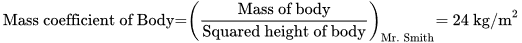### Molecular Biology and Genetics

Example 2
Generic abbreviated
NPU54759 U—Glycosaminoglycans/Creatininium; mass coefficient(mass/am.s.; proc.) = ? g/mol
Full result NPU54759 Urine—Glycosaminoglycans/Creatininium; mass coefficient(mass/amount-of-substance; proc.) = 1.4 gram per mol
Abbreviated result NPU54759 U—Glycosaminoglycans/Creatininium; mass coefficient(mass/am.s.; proc.) = 1.4 g/mol
Written expression The mass coefficient of Mr. Smith’s Glycosaminoglycans/Creatininium in Mr. Smith’s urine is [1.4] g/mol. The calculation is the division of mass of Glycosaminoglycans divided by amount-of-substance of Creatininium.
Equation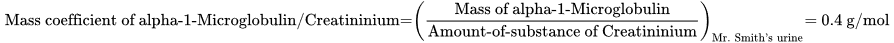### Clinical Toxicology

Example 3
Generic abbreviated
NPU29339 U—11-Nor-delta(9)-tetrahydrocannabinol-9-carboxylic acid/Creatininium; mass coefficient(mass/am.s.; proc.) = ? mg/mol
Full result NPU54757 Urine—11-Nor-delta(9)-tetrahydrocannabinol-9-carboxylic acid/Creatininium; mass coefficient(mass/amount-of-substance; procedure) = 34 mg/mol
Abbreviated result NPU29339 U—11-Nor-delta(9)-tetrahydrocannabinol-9-carboxylic acid/Creatininium; mass coefficient(mass/am.s.; proc.) = 34 mg/mol
Written expression The mass coefficient of Mr. Smith’s 11-Nor-delta(9)-tetrahydrocannabinol-9-carboxylic acid/Creatininium in Mr. Smith’s urine is  mg/mol. The calculation is the division of mass of 11-Nor-delta(9)-tetrahydrocannabinol-9-carboxylic acid divided by amount-of-substance of Creatininium.
Equation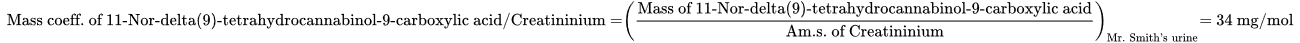### Reference

NPU database, https://www.ifcc.org/ifcc-scientific-division/sd-committees/c-npu/npusearch/ and http://www.labterm.dk/default.aspx
• # Kind-of-property

• ## Kind-of-quantity

### Reference

• Dybkaer R. Concept system on ‘quantity’: formation and terminology. Accredit Qual Assur. 2013;18(3):253-60.
• Dybkaer R. ISO terminological analysis of the VIM3 concepts ‘quantity’ and ‘kind-of-quantity’. Metrologia. 2010;47(3):127-34.
Date Term Note
2015-03-19 Mass coefficient Term established

QU80257